# Manifest (spin 12)

``````---+-----+-----
1 | {1} | {2}
---+-----+-----
2 |  3  | 101
---+-----+-----
3 |{102}| 111
---+-----+-----
``````

There are some mathematical shape of this residual objects. Torus is basically a donut shape, which has the property of of having variable Gaussian curvature. Some parts of the surface has positive curvature, others zero, others negative.

The blue parts of the torus above have positive curvature, the red parts negative and the top grey band has zero curvature. If our 3 dimensional space was like the surface areas of a 4 dimensional torus, the parts would have different angle sums.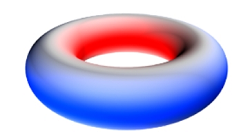These are two more bizarre shapes with strange properties. Mobius strip only has one side, if you start anywhere on its surface and follow the curvature round you will eventually return to the same place having travelled on every part of the surface.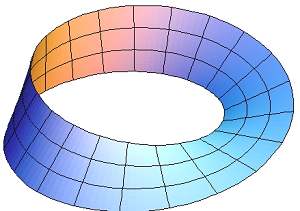The Klein bottle is in someways a 3D version of the Mobius strip and even though it exists in 3 dimensions, to make a true one you need to "fold through" the 4th dimension.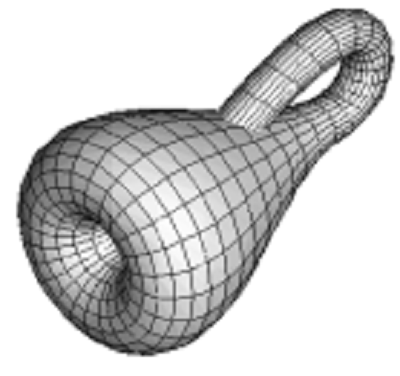By our project, this partition stands as the prime identity. The tabulation below shows the 2nd prime identity where the 20 out of the largest part = 21 goes to rank = 10 via crank = 20-11 = 9. These 10 and 9 are associated with the 19th prime identitity.

``````           largest part = 21 → 11+13+12 = 36  →  MEC30
↓                      |
---+-----+-----+-----+-----+                   ↓
1 | 19  | 1   | 20  | 21  |-------------------|-----
---+-----+-----+-----+-----+                   ↓     |
2 | 18  | 21  | 39  | 60  |-------------------      |
---+-----+-----+-----+-----+                   |     |
3 |{63} | 40  | 103 | 143 |-------------      |     |
---+-----+-----+-----+-----+             |     |     |
4 | 37  | 104 | 141 | 245 |-------      |     |     |
---+-----+-----+-----+-----+       |     |     |     |
5 | 10* | 142 | 152 | 294 |- 11** | 13  | 12  | 12  | 18
---+-----+-----+-----+-----+       |     |     |     |
6 | 24  | 153 | 177 | 332 |-------      |     |     |
---+-----+-----+-----+-----+             |     |     |
7 | 75  | 178 | 253 | 431 |-------------      |     |
---+-----+-----+-----+-----+                   |     |
8 | 30  | 254 | 284 | 538 |-------------------      |
---+-----+-----+-----+-----+                   ↓     |
9 | 1   | 285 | 286 | 571 |-------------------|-----
===+=====+=====+=====+=====+                   ↓
45 | 277 |                      ← 11+13+12=36 ←  MEC30
---+-----+                                     |
↑
Note:
10* stands as the central rank
11** stands as the central parts
``````

In order to describe real physical phenomena using string theory, one must therefore imagine scenarios in which these extra dimensions would not be observed in experiments so it would become the irrational partitions.

Mapping the quantum way within a huge of primes objects (5 to 19) by lexering (11) the ungrammared feed (7) and parsering (13) across syntax (17) (₠Quantum).

``````\$True Prime Pairs:
(5,7), (11,13), (17,19)

layer|  i  |   f
-----+-----+---------
|  1  | 5
1  +-----+
|  2  | 7
-----+-----+---  } 36 » 6®
|  3  | 11
2  +-----+
|  4  | 13
-----+-----+---------
|  5  | 17
3  +-----+     } 36 » 6®
|  6  | 19
-----+-----+---------

layer | node | sub |  i  |  f
------+------+-----+----------
|      |     |  1  | -------------------- _site ---  71 = 72-1
|      |  1  +-----+                        |
|  1   |     |  2  | (5)                  _saas
|      |-----+-----+                        |
|      |     |  3  | ---------            _data
1   +------+  2  +-----+----      |             |
|      |     |  4  |         5x ---       _posts
|      +-----+-----+          |     |       |
|  2   |     |  5  | (7) -----      |     _drafts
|      |  3  +-----+                |       |
289+11=300   |     |  6  |                |     _plugins
------+------+-----+-----+----- 72 x 6   7x ------------ 11x = 77 (rational)◄--
|      |     |  7  |                |     _includes                      |
|      |  4  +-----+                |       |                            |
|  3   |     |  8  | (11)  ---      |     _layouts                       |
|      +-----+-----+          |     |       |                            |
|      |     |  9  |         2x ---        assets  (69 = 72-3)           |
2   +------|  5  +-----+-----     |             |                            |
|      |     |  10 | ---------            _saas                          |
|      |-----+-----+                        |                            |
|  4   |     |  11 | (13) ----------------_site --  71 = 72-1            |
|      |  6  +-----+                                                     |
329+71=400   |     |  12 |------------------------------  70 = 72-2            |
------+------+-----+-----+                                                    11x
|      |     |  13 |                                                     |
|      |  7  +-----+                                                     |
|  5   |     |  14 | (17) ◄------------------------------------------- (17)
|      |-----+-----+                                                     |
|      |     |  15 | ◄-- 42 x 6 partitions of 13 (irrational)            |
3   +------+  8  +-----+-----                                                +
|      |     |  16 |                                                     |
|      |-----+-----+                                                     |
|  6   |     |  17 | (19) ◄------------------------------------------- (19)
|      |  9  +-----+                                                     |
168+32=200   |  |  |  18 |------------------------------  68 = 72-4            |
------|------|--|--+-----+                                                     |
900 -----                                                               |
|
Tabulate Prime by Power of 10                                                  |
loop(10) = π(10)-π(1) = 4-0 = 4                                            (36)
loop(100) = π(100)-π(10)-1th = 25-4-2 = 19                                   |
loop(1000) = π(1000) - π(100) - 10th = 168-25-29 = 114                       |
|
-----------------------+----+----+----+----+----+----+----+----+----+-----   |
True Prime Pairs Δ    |  1 |  2 |  3 |  4 |  5 |  6 |  7 |  8 |  9 | Sum    |
=======================+====+====+====+====+====+====+====+====+====+=====   |
19 → π(10)            |  2 |  3 |  5 |  7 |  - |  - |  - |  - |  - | 4th  (19)
-----------------------+----+----+----+----+----+----+----+----+----+-----   +
17 → π(20)            | 11 | 13 | 17 | 19 |  - |  - |  - |  - |  - | 8th  (17)
-----------------------+----+----+----+----+----+----+----+----+----+-----
13 → π(30) → 12 (Δ1)  | 23 | 29 |  - |  - |  - |  - |  - |  - |  - |10th    |
=======================+====+====+====+====+====+====+====+====+====+=====   |
11 → π(42)            | 31 | 37 | 41 |  - |  - |  - |  - |  - |  - |13th   11x
-----------------------+----+----+----+----+----+----+----+----+----+-----
7 → π(60) → 19 (Δ12) | 43 | 47 | 53 | 59 |  - |  - |  - |  - |  - |17th
-----------------------+----+----+----+----+----+----+----+----+----+-----
5 → π(72) → 18 (Δ13) | 61 | 67 | 71 |  - |  - |  - |  - |  - |  - |20th
=======================+====+====+====+====+====+====+====+====+====+=====
3,2 → 18+13+12 → 43  | 73 | 79 | 83 | 89 | 97 | 101| 103| 107| 109|29th
=======================+====+====+====+====+====+====+====+====+====+=====
Δ                                                            Δ
12+13+(18+18)+13+12   ← 36th-Δ1=151-1=150=100+2x(13+12)   ←   30th = 113 = 114-1

``````

Within a cycle this scheme would generate the prime platform which is performing the rank of 10 shapes starting with the primes 2,3,5,7. Via the 11 partitions as the lexer and 13 frames as the parser we do a recombination to build the grammar in 6 periods.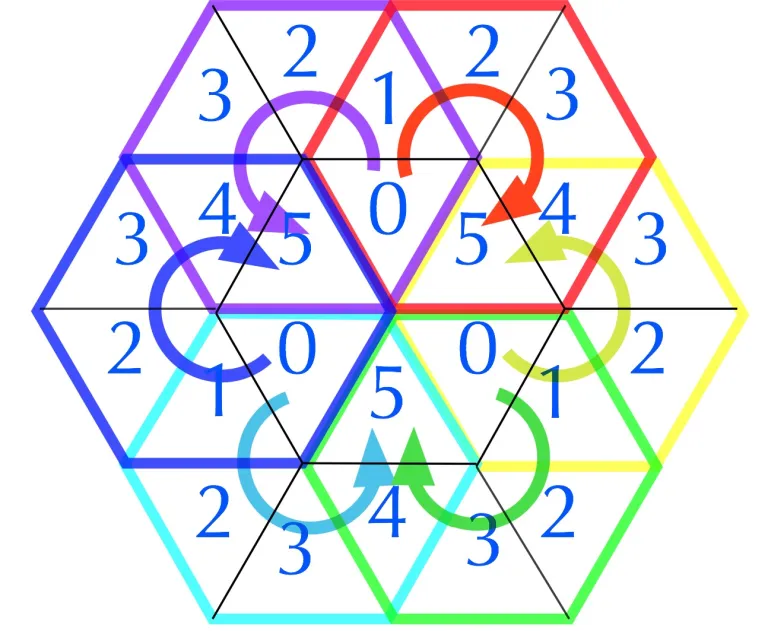For starters, it possesses a perfectly balanced structural and numeric symmetries. So it conforms the intervals between the prime roots of the Prime Spiral Sieve (and every subsequent row or rotation of the sieve) with the period eight (8) difference sequence.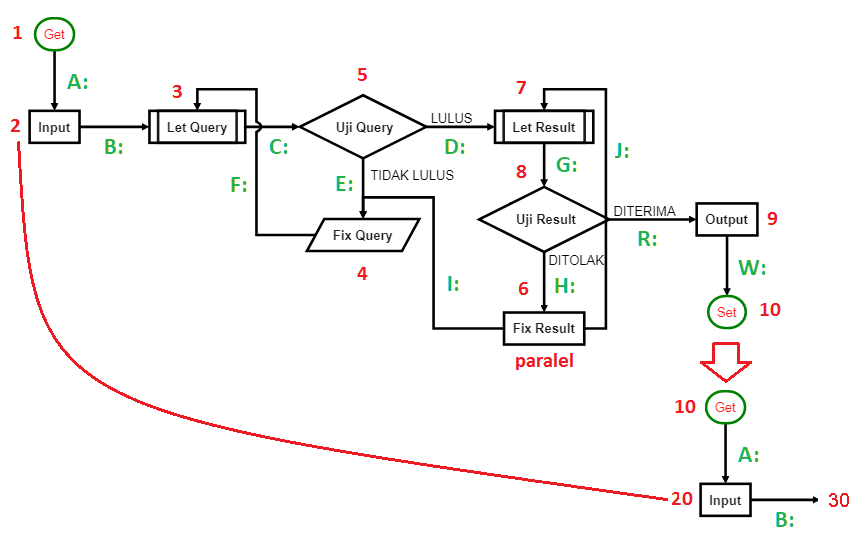These two (2) exponent are acting as the exchange zones of the MEC30 behaviour of the other two operators. This interactions is managed in between 4 identities of (26 to 29) and 2 identities (30 to 31) by means of Triangular waves.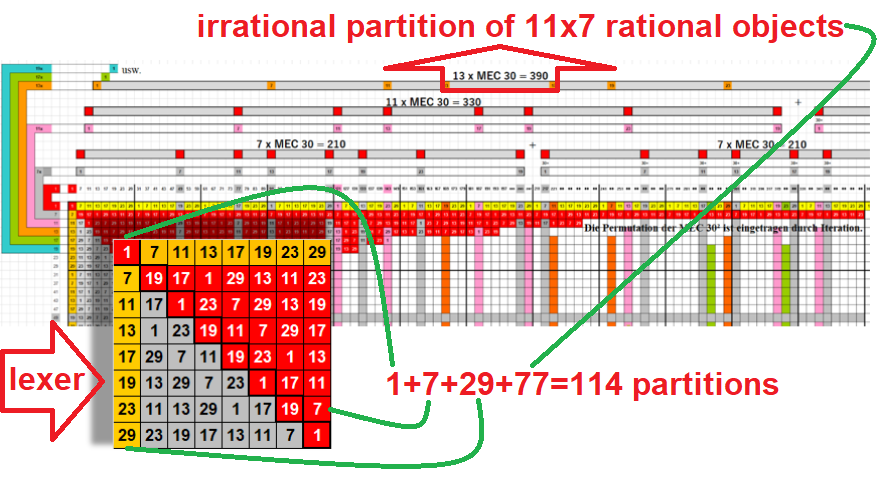When the subclasses of partitions are flatten out into a matrix, you want to take the Jacobian of each of a stack of targets with respect to a stack of sources, where the Jacobians for each target-source pair are independent .

It's possible to build a Hessian matrix for a Newton's method step using the Jacobian method. You would first flatten out its axes into a matrix, and flatten out the gradient into a vector (Tensorflow).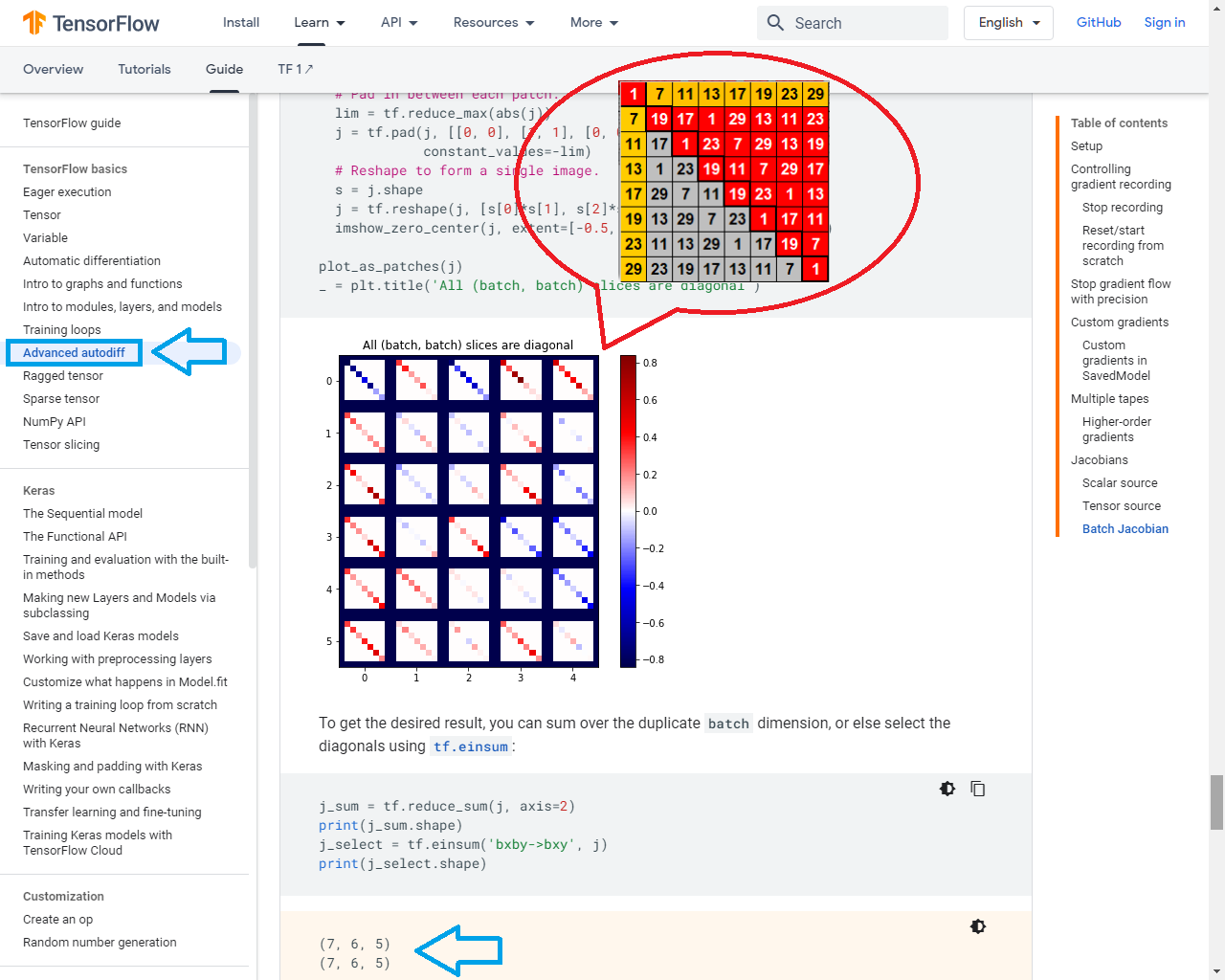By The GitHub Runner you can connect to the Google COS Instance. For self-hosted runners defined at the organization level, configure runs-on.group in your workflow file to target a runner groups or combine groups and labels.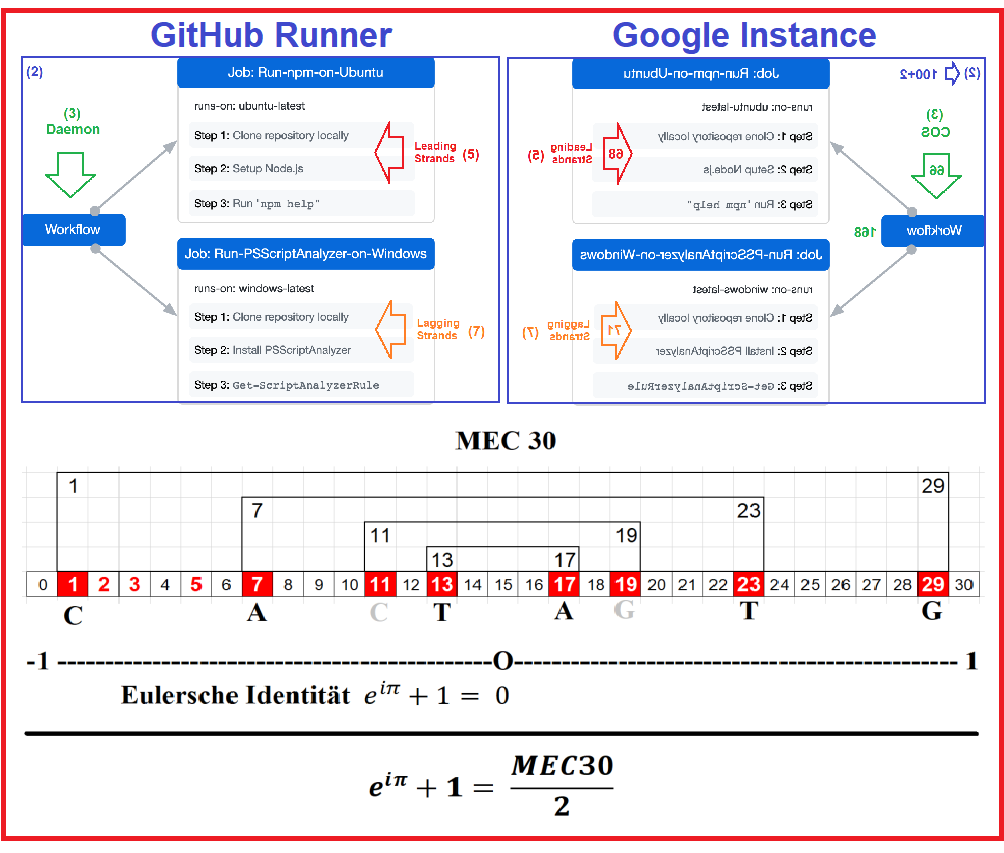A seemingly unrelated construction is the j-function of number theory. This object belongs to a special class of functions called modular functions, whose graphs form a certain kind of repeating pattern.

Although this function appears in a branch of mathematics that seems very different from the theory of finite groups, the two subjects turn out to be intimately related (Wikipedia).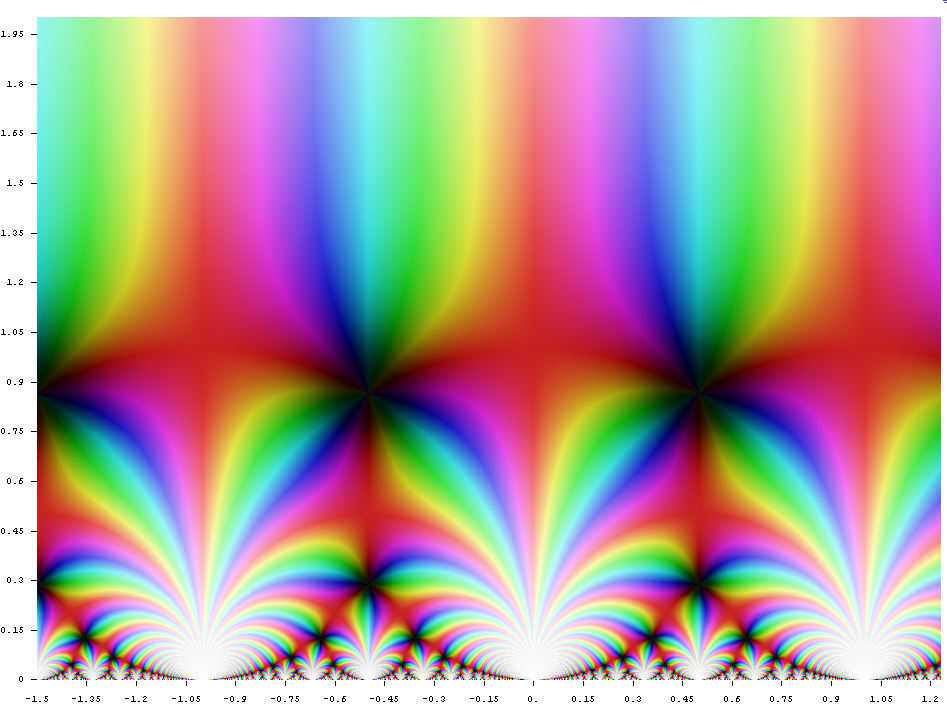We propose a new higher dimensional version of the McKay correspondence which enables us to understand the Hodge theory assigned to singular Gorenstein varieties by physicists, and so-called Higgs bundles.

Hodge theory can be extended to cohomology with coefficients in nonabelian groups between flat vector bundles which, by the Riemann-Hilbert correspondence, are the same as local systems (Hodge Theory in String Theory)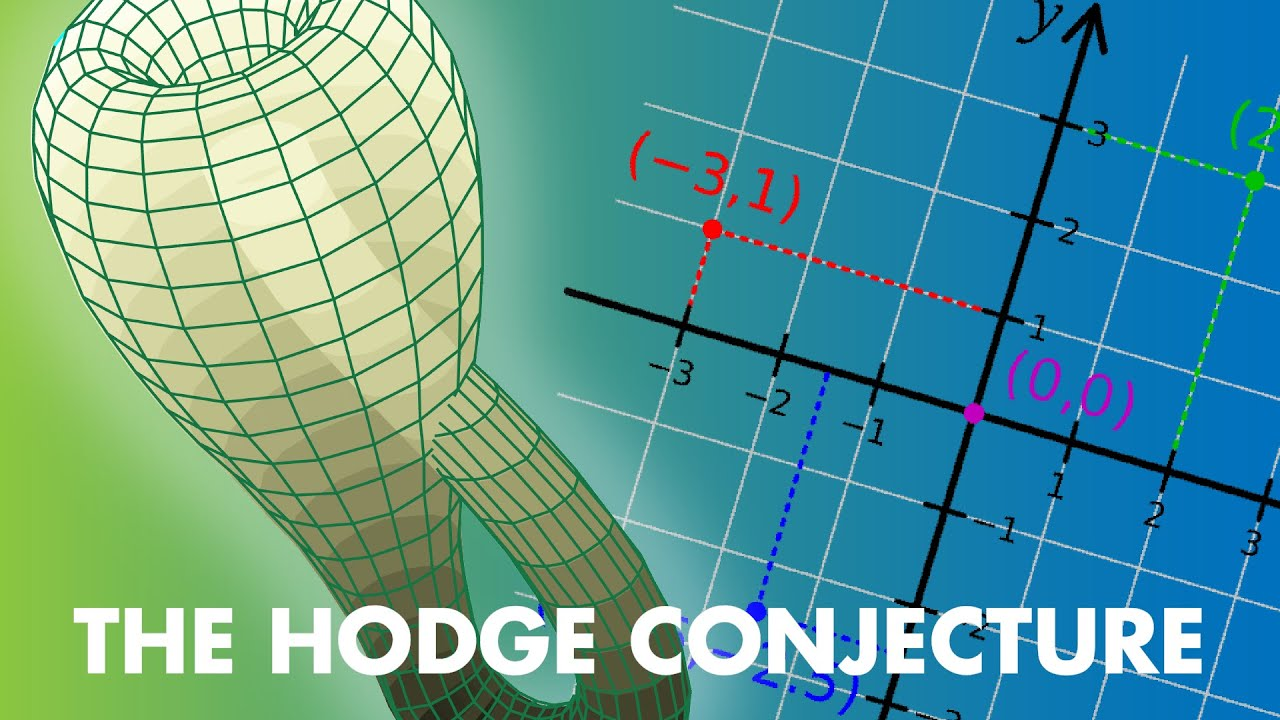Our results lead to the conjecture that string theory indicates the existence of some new cohomology theory for algebraic varieties with Gorenstein singularities.